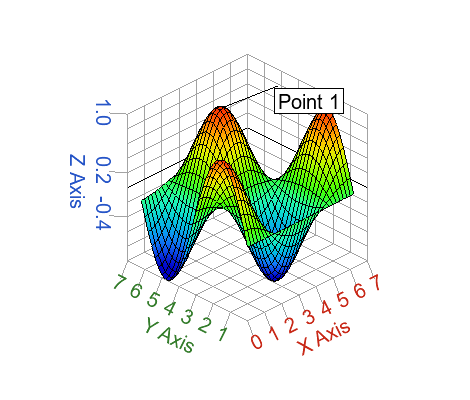# text

Creates a textbox at coordinate x,y with text 't'.

## Syntax

h = text(x, y, t)

h = text(x, y, z, t)

h = text(..., property, value, ...)

## Inputs

x, y, z
x, y, and z coordinates for the textbox. z is valid only in 3D plots.
Type: double | integer
Dimension: scalar
t
Text to be added in the axes.
Type: string
Dimension: scalar
property
Properties that control the appearance or behavior of the graphics object.
Type: string
Dimension: scalar
value
Value of the properties.
Type: double | integer | string
Dimension: scalar

## Outputs

h
Handle of the text graphics object.

## Example

Simple textbox example:
clf
text(1,1,'hello world')
text example with alignment properties:
clf;
scatter(1, 1);
t = text(1,1,'hello world', 'horizontalalignment','center', 'verticalalignment', 'top');
text example in a 3D plot:
clf;
x=[0:0.2:2*pi];
y=x;
z=sin(x')*cos(y);
s=surf(x, y, z);
t = text(10,90,'hello world');
text pointing on a 3D point example:
clf;
x=[0:0.2:2*pi];
y=x;
z=sin(x')*cos(y);
s=surf(x, y, z);
t = text(3.2,4.6,1,'Point 1');